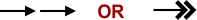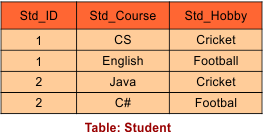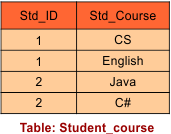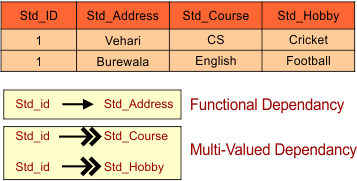Select Page

# Fourth Normal Form (4NF)

According to fourth normal form (4NF), A relation will be in 4NF if,

It is in BCNF form and has no multi-valued dependency.

#### Multivalued Dependency

• For a single value of A, More than one values (either similar or not) of B exists.
• Multivalued dependency must contains at least 3 attributes (i.e. A, B,C) Because In the Multivalued dependency, two attributes (i.e. B,C) in a table are independent to each other, but both attributes (B,C) depend on a third attribute (i.e. A)

## Notation of Multi-Valued DependencyExample: Consider the Student Table as given below.Above table does not  holds the conditions of 4NF because it Holds the Multivalued Dependency.

Explanation

• FOR std_id =1 there are two values of Std_course (i.e. CS and English) and same for Std_id =2 there are two values of Std_course (i.e. Java and C#).
• Columns “Std_course” and “Std_hobby” are independent to each other but depend on “Std_id”. Atleast three values also exist in the given table.

Above both points tells that Multivalued Dependency exist in table. As we know if there exist Multivalued Dependency then that table is not in 4NF.

## How To Satisfy 4th Normal Form?

To remove 4NF problem and satisfy the 4NF, we can decompose the “Student” table as given below

Table 01 “Student_Course”Table 02 “Student_Hobbies”As we see table is also in 4NF as for each value of column “A” more than one values of column “B” exist. Now this relation satisfies the 4NF.

## Functional Dependency And Multi-Valued Dependency

A table can also have functional dependency (FD) along with multi-valued dependency as given below.These problems can easily resolve by table decomposition.

Help Other’s By Sharing…